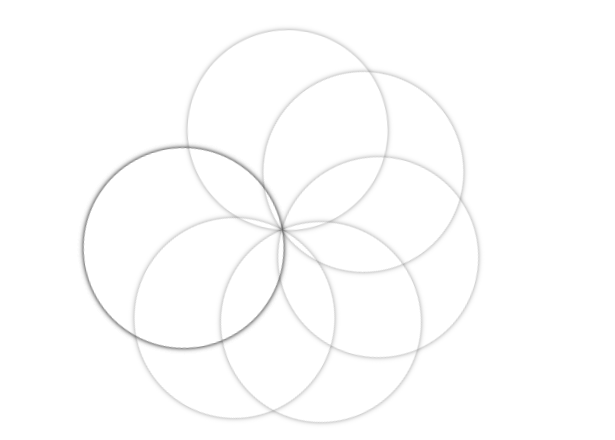GeeksforGeeks App
Open AppBrowser
Continue

# How to create rotating disc effect using CSS ?

The Rotating Disc Effect also known as Overlapping Disc Effect is a type of illusion effect that can be used for various purposes on a website. It can be used in anything from a loader sticker to creating an illusion for the user. It is called overlapped disc because there are many overlapped discs which are rotating around a single point.

Approach: The approach is to create all the discs first and then use keyframes and n-th child properties to rotate them.

HTML Code: In this section, we have created a un-ordered list(ul) with list-items(li) inside it. The number of list-items is equal to the number of discs.

 ```<``html` `lang``=``"en"``>``  ``<``head``>``    ``<``meta` `charset``=``"UTF-8"` `/>``    ``<``meta` `name``=``"viewport"` `content``=``        ``"width=device-width, initial-scale=1.0"` `/>``    ``<``title``>Rotating Disc Effect`` ````  ``<``body``>``    ``<``ul``>``      ``<``li``>``      ``<``li``>``      ``<``li``>``      ``<``li``>``      ``<``li``>``      ``<``li``>``      ``<``li``>``      ``<``li``>``    ````  `````

CSS Code: For CSS, follow the given steps.

• First thing is to create disc by using the border-radius property. Set the border-radius to 50% to create a perfect circle.
• Now, use animation with an identifier to be used later with keyframes. We have used animate as our identifier.
• Now, use keyframes to rotate the disc for each frames. Here we have used 0deg for first frames and 359deg for second frame.
• Now, use n-th child property to apply a delay of 1st to each disc. It helps to rotate each disc with some delay that is responsible for the illusion kind of effect.

Tip: You can apply different opacity colors to each disc to make it look more appealing.

 ``

Complete Code: It is the combination of the above two sections of code.

 ```<``html` `lang``=``"en"``>``  ``<``head``>``    ``<``meta` `charset``=``"UTF-8"` `/>``    ``<``meta` `name``=``"viewport"` `content``=``        ``"width=device-width, initial-scale=1.0"` `/>``    ``<``title``>Rotating Disc Effect``    ``<``style``>``      ``ul {``        ``margin: 0;``        ``padding: 0;``        ``position: absolute;``        ``left: 40%;``        ``top: 20%;``      ``}`` ` `      ``ul li {``        ``list-style: none;``        ``width: 200px;``        ``height: 200px;``        ``position: absolute;``        ``top: 0;``        ``border-radius: 50%;``        ``box-shadow: 0 0 5px rgba(0, 0, 0, 0.5);``        ``animation: animate 7s linear infinite;``        ``transform-origin: bottom center;``      ``}`` ` `      ``@keyframes animate {``        ``0% {``          ``transform: rotate(0deg);``        ``}``        ``100% {``          ``transform: rotate(359deg);``        ``}``      ``}`` ` `      ``ul li:nth-child(1) {``        ``animation-delay: 0s;``      ``}``      ``ul li:nth-child(2) {``        ``animation-delay: 1s;``      ``}``      ``ul li:nth-child(3) {``        ``animation-delay: 2s;``      ``}``      ``ul li:nth-child(4) {``        ``animation-delay: 3s;``      ``}``      ``ul li:nth-child(5) {``        ``animation-delay: 4s;``      ``}``      ``ul li:nth-child(6) {``        ``animation-delay: 5s;``      ``}``      ``ul li:nth-child(7) {``        ``animation-delay: 6s;``      ``}``      ``ul li:nth-child(7) {``        ``animation-delay: 7s;``      ``}``    ````  ````  ``<``body``>``    ``<``ul``>``      ``<``li``>``      ``<``li``>``      ``<``li``>``      ``<``li``>``      ``<``li``>``      ``<``li``>``      ``<``li``>``      ``<``li``>``    ````  `````

Output:My Personal Notes arrow_drop_up
Related Tutorials